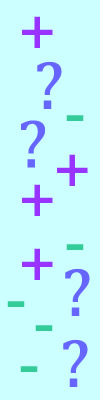#### You may also like### Prompt Cards

These two group activities use mathematical reasoning - one is numerical, one geometric.### Consecutive Numbers

An investigation involving adding and subtracting sets of consecutive numbers. Lots to find out, lots to explore.### Exploring Wild & Wonderful Number Patterns

EWWNP means Exploring Wild and Wonderful Number Patterns Created by Yourself! Investigate what happens if we create number patterns using some simple rules.

# Sums and Differences 2

##### Age 7 to 11Challenge LevelThis challenge is about adding and subtracting two-digit numbers.

Pick two two-digit numbers.
Find the sum of the numbers.
Find the difference between the numbers.
Then find the sum and difference of the sum and difference.

What do you notice about the numbers?
Does this always happen?
Try for other starting numbers.

For example:
My numbers are 24 and 31.
The sum of them is 55 and the difference between them is 7.
Now my numbers are 55 and 7.
The sum of them is 62 and the difference between them is 48.